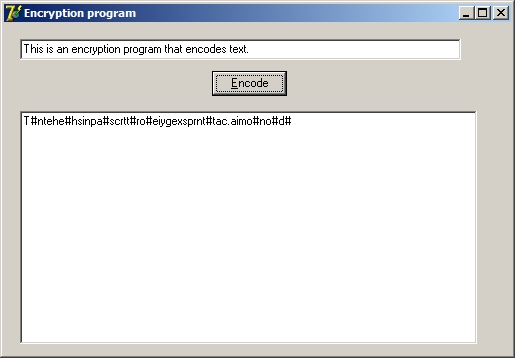DELPHI: Encryption examples

1) Basic encryption changing ordinal value of characters

Convert text using the ORD function that gives the ordinal (integer) value of any ASCII character add 4 to this value and write back the character using the CHR function (that returns the ASCII character for a number).

For example the character 'a' would become 'e', 'b' would become 'f' - characters are replaced with the character four places on:

 a b c d e f g h i j k l m n o p q r s t u v w x y z e f g h i j k l m n o p q r s t u v w x y z { ¦ } ~

The ordinal value of 'a', which is 97, is increased to 101 (the ordinal value of 'e').

A possible solution for this program where the values are converted by increasing the ordinal value of each character by four and then converting it back to the original text would be:procedure TForm1.Button1Click(Sender: TObject);
var
sInput : String;
iCount, iLength : Integer;
begin
sInput := Edit1.Text;
iLength := Length(sInput);
form1.caption := chr(101);
FOR iCount := 1 to iLength do
begin
sInput[iCount] := chr(ord(sInput[iCount])+4);  //Encoding
end;
Label1.caption := sInput;  //Display encoded text

//Decoding section
//This will probably be placed in another procedure.

FOR iCount := 1 to iLength do
begin
sInput[iCount] := chr(ord(sInput[iCount])-4);
end;
Label2.caption := sInput;
end;

end.

2) Encryption with text in a two dimensional array

Calculate the length of a message and round up to the next multiple of ten then write out the message in rows of ten characters. The message is then read in columns from left to right to create the encoded text.

The hash (#) replaces all spaces and fills up the end of the text up to a multiple of ten.

For example the text: "This is an encryption program that encodes text." will be represented as:

 T h i s # i s # a n # e n c r y p t i o n # p r o g r a m # t h a t # e n c o d e s # t e x t . # #

This text consists out of 48 characters (with spaces in between) therefore two hashes must be added at the end to round it off to 50. To get the encoded message the text is then read from top to bottom and then from left to right.

The encoded text should then read:
T#ntehe#hsinpa#scrtt#ro#eiygexsprnt#tac.aimo#no#d#

A possible solution for this example is:var
Form1: TForm1;
arrMessage : array[1..10,1..100] of char; //2D array

implementation

{\$R *.dfm}

procedure TForm1.Button1Click(Sender: TObject);
var
sInput, sEncode : String;
iCount, iLength, iHashNeed, iRow, iCol, iTotal, iWCount : Integer;

begin
RichEdit1.Clear;
iHashNeed := 0;
sInput := Edit1.Text;
iLength := Length(sInput);
if iLength MOD 10 <> 0 then //Determine if # needed
begin
iHashNeed := (((iLength DIV 10) + 1) * 10) - iLength;
FOR iCount := 1 to iHashNeed DO
sInput := sInput + '#'
end;

FOR iCount := 1 to iLength DO //Change spaces to #
begin
if sInput[iCount] = ' ' then
sInput[iCount] := '#';
end;

iTotal := length(sInput) DIV 10;

iWCount := 0;

//Message entered into 10 columns
FOR iRow := 1 to iTotal do
begin
FOR iCol := 1 to 10 do
begin
iWCount := iWCount + 1;
arrMessage[iCol, iRow] := sInput[iWCount];
end;
end;

//Reads message and displays it in a single line
sEncode := '';
FOR iCol := 1 to 10 do
begin
FOR iRow := 1 to iTotal do
begin
sEncode := sEncode + arrMessage[iCol, iRow]
end;
end;

end;

end.

Sample Exercises

a) Use example 2 and create a program that uses a created function to encode the inserted text. The user must also be able to specify the amount of columns used.

b) Create a program with which you can specify a text file and a new a file name and the program then encodes the message in the new file.